• %% **************有向图的关联矩阵和邻接矩阵******************** % 输入参数：F 邻接矩阵或关联矩阵 f转换参数，0表示邻接矩阵转换为关联矩阵 // % 1表示关联矩阵转换为邻接矩阵 // ...
%% **************有向图的关联矩阵和邻接矩阵********************
% 输入参数：F 邻接矩阵或关联矩阵 f转换参数，0表示邻接矩阵转换为关联矩阵      //
% 1表示关联矩阵转换为邻接矩阵                                                                    //
% 输出: W 转换所得矩阵                                                                               //
%%
function W = mattransf(F, f)

if f == 0                                             %邻接矩阵转换为关联矩阵
m = sum(sum(F));
n = size(F);
W = zeros(n,m);
k = 1;
for i =1:n
for j = 1:n
if F(i,j)~=0 && j>i
W(i,k) = 1;                           %关联矩阵始点值为1
W(j,k) = -1;                          %关联矩阵终点值为-1
k= k+1;
end
end
end
elseif f == 1                                        %关联矩阵转换为邻接矩阵
m = size(F,2);
n = size(F,1);
W = zeros(n);
for i = 1:m
a = find(F(:,i)~=0);
if F(a(1),i) == 1
W(a(1),a(2)) = 1;
else
W(a(2),a(1)) = 1;
end
end
else
fprint('Please input the right value of f');
end
展开全文function input
• 关联矩阵的值为1时，有向边由a1指向a2 当关联矩阵的值为-1时，有向边由a2指向a1 程序参数说明 当f=0时，邻接矩阵转换为关联矩阵，F表示邻接矩阵，W表示关联矩阵 当f=1时，关联矩阵转换为邻接矩阵，F表示...
算法思想
1.邻接矩阵转换为关联矩阵
如果邻接矩阵的值不为0，则关联矩阵的始点赋值为1，终点赋值为-1
2.关联矩阵转化为邻接矩阵
找出每一列关联矩阵的值不为0的两个下标a1，a2
当关联矩阵的值为1时，有向边由a1指向a2
当关联矩阵的值为-1时，有向边由a2指向a1
程序的参数说明
当f=0时，邻接矩阵转换为关联矩阵，F表示邻接矩阵，W表示关联矩阵
当f=1时，关联矩阵转换为邻接矩阵，F表示关联矩阵，W表示邻接矩阵
MATLAB实现
function W = mattransf(F, f)
if f == 0
m = sum(sum(F));
n = size(F,1);
W = zeros(n, m);
k = 1;
for i = 1 : n
for j = i : n
if F(i,j) ~= 0;
W(i,k) = 1;
W(j,k) = -1;
k = k + 1;
end
end
end
end

if f == 1
m = size(F,2);
n = size(F,1);
W = zeros(n,m);
for i = 1 : m
a = find(F(:,i) ~= 0);
if find(a(1),i) == 1
W(a(1),a(2)) = 1;
else
W(a(2),a(1)) = 1
end
end
end
W;

测试
测试用例1：邻接矩阵
F = [0 0 0 1 0 1; 1 0 1 0 0 1; 0 0 0 1 1 0; 0 0 0 0 0 0; 0 0 0 1 0 0; 0 0 0 0 1 0];
测试结果1：W =
 1     1     0     0     0     0     0     0     0
0     0     1     1     0     0     0     0     0
0     0    -1     0     1     1     0     0     0
-1     0     0     0    -1     0     0     0     0
0     0     0     0     0    -1     0     0     0
0    -1     0    -1     0     0     0     0     0

测试用例2：关联矩阵
F = [1 1 1 0 0 0; -1, 0 0 1 1 0; 0 -1 0 -1 0 1; 0 -1 0 -1 0 1; 0 0 -1 0 -1 -1];
测试结果2：W =
 0     1     1     1
0     0     1     1
0     0     0     1
0     0     0     0



展开全文• 《大学离散数学》图的矩阵表示，无向图关联矩阵有向图关联矩阵 无向图关联矩阵相关计算 mport numpy as np ramdom_matrix = np.array([[1,1,1,0,0,0], [0,1,1,0,1,0], [0,0,0,1,1,0], ...
《大学离散数学》图的矩阵表示，无向图关联矩阵，有向图关联矩阵
无向图关联矩阵相关计算
mport numpy as np
ramdom_matrix = np.array([[1,1,1,0,0,0],
[0,1,1,0,1,0],
[0,0,0,1,1,0],
[1,0,0,1,0,2]])#输入你想算的矩阵
print("每条边关联顶点个数")
print(sum(ramdom_matrix))
a = 0
for i in range (0,4):
print("第",i,"行元素的度数为")
print(sum(ramdom_matrix[i]))
a = a + sum(ramdom_matrix[i])
print("根据握手定律，可知顶点度数总和为：")
print(a)

有向图邻接矩阵
import numpy as np
ramdom_matrix = np.array([[1,2,1,0],
[0,0,1,0],
[0,0,0,1],
[0,0,1,0]])#输入需要算的矩阵
print("每列元素的入度为{}".format(sum(ramdom_matrix)))

a = 0
for i in range (0,4):
print("第",i,"行元素的出度为{}".format(sum(ramdom_matrix[i])))
a = a + sum(ramdom_matrix[i])
'''矩阵乘法'''
print("\n")
A_square = np.dot(ramdom_matrix,ramdom_matrix)
print("A²为\n",A_square)
A_cube = np.dot(ramdom_matrix,A_square)
print("A³为\n",A_cube)
A_quartic = np.dot (A_square,A_square)
print("A四次方为\n",A_quartic)
print("\n从vi到vj长度小于等于l的通路数或回路数为")
B = ramdom_matrix + A_square + A_cube + A_quartic
print(B)


虽说是无聊写的代码，但这个代码我感觉把我带向了喜欢编程的行列中。本人学生党，第一次发博客，很多不足，望读者可以体谅，希望以后可以做个真正的程序员！RushB!!!


展开全文python 线性代数 算法
• MATLAB源码集锦-有向图关联矩阵和邻接矩阵相互转换算法代码
• 根据邻接矩阵“mAdj”返回稀疏关联矩阵“mInc”。 关联矩阵边排序是根据从第一... 如果有向的关联矩阵 mInc 包含 -1s，表示“进入”边缘，1s 表示“离开”边缘。 如果该是无向，则入射矩阵mInc仅包含1s。matlab
• ## 图 网络 关联矩阵

千次阅读 2016-10-10 16:31:40
在实际应用中，可以给边加上箭头来表示电流流向，这就是一个有向图： 然后我们可以定义一个关联矩阵(Incidence Matrices)，这个矩阵描述如下：列代表结点，行代表边。如果从结点a到结点b有一条边（箭尾是a，...
一、下面就是一个简单的图（在离散数学中称之为“图”），图有两个元素：结点(nodes)，边(edges)。在实际应用中，可以给边加上箭头来表示电流的流向，这就是一个有向图：然后我们可以定义一个关联矩阵(Incidence Matrices)，这个矩阵的描述如下：列代表结点，行代表边。如果从结点a到结点b有一条边（箭尾是a，箭头是b），那么就把a置为-1，把b置为1,。根据这个准则，上图所生成的关联矩阵如下：在图2中我们可以看到结点1， 2，3和边1,2,3形成了一个封闭的回路(loop)。该loop的关联矩阵如下:这个矩阵很有趣，因为它的第三行是第一行与第二行的和，这说明该矩阵的行是线性相关的，从图中我们也可以看到边1和边2的和为3（有点像向量的加法）。
矩阵A的零空间（nullspace）可以通过Ax = 0求得，过程如下：当x1 = x2 = x3 = x4时，Ax = 0，所以矩阵dim( N(A) ) = 1。所以rank(A) = n - dim( N(A) ) = 4 - 1 = 3。现在我们考虑A的左零空间N(AT)，AT y=0。AT =AT y =我们如何求解N(AT)呢，Strang教授给了我们一个很直观的方法，首先，我们把上边等式的左边的两个矩阵相乘的结果写出来，得到：
（-y1） + （-y3） + （-y4） = 0
y1 - y2 = 0;
y2 + y3 - y5 = 0;
y4 + y5 = 0.
我们还是回到上面那个有向图，来仔细观察下，发现y1， y3 ， y4正是流入（流入为0）和流出结点1的电流之和，这就是我们熟悉的基尔霍夫电流定律，另外的三个等式可以相同得出。所以，我们就可以根据loop数来确定A的左零空间的基（没有loop的图称为树(tree)），我们有两个相互独立的loop(结点：1-2-3和1-3-4)，当然还有一个大的loop(1-2-3-4)，但是我们可以通过两个子loop相加得到，所有，基这样找：
y1 = 1, y2 = 1, y3 = -1 => y4 = 0, y5 = 0，另外一个基：
y3 = 1, y4 = -1, y5= -1 => y1 = 0, y2 = 0。
我们在选取y1~y5的时候，应当使结果只含有1， -1， 0这三个值。所以dim( N(AT) ) = 2，rank(A) = 5 - dim( N(AT) ) = 3。与上面的结论一致。
这里有个小结论：dim( N(AT) )是回路的数，5是A的列数，AT的行数，也是边数，r是A的秩，也等于结点数-1（这个不是偶然，可以再举其他的图试一下）。所以我们又以下结论：
#loops = #edges - (#nodes - 1)，也可以写成：
#loops - #edges + nodes = 1，这就是我们熟悉的欧拉公式
似乎是说到结尾了，但是还有一点Strang教授提醒我们观察：如果我们把结点之间的差看作是电势差e，那么Ax = e，并且利用欧姆定律有y = Ce，且我们还有基尔霍夫定律AT y = 0，并且我们给上面的图外加一个电流源（电压源），有AT y = f，把这些式子整合到一起，得到AT C Ax = f，B = AT C A，那么矩阵B就是一个对称阵(Symmetric Matrix)。这有什么用呢，当我们求解trace(AT
A)时就会发现一个更加有趣的现象，我只大致说一下，具体过程笔算就可以了：
trace(AT A)就是AT A的对角线的元素之和，然而A, AT的各个元素的绝对值不是1就是0，那么AT A的对角线元素之和就是A的各列的元素之和（可以设一个任意矩阵算一下就知道了）。所以trace(AT A) = 3 + 2 + 3 + 2 = 10，这个10是什么呢，我们再回到那个有向图，3是结点1的度（入度和出度之和），2是结点2的度。。。所以10就是所有结点的度之和。

二、矩阵A的列空间（column space）
假设A=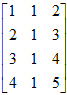，则A的列空间C(A)是R4的子空间，C(A)中是A中列的线性组合，下面我们将列空间与线性方程组联系起来，以更好的认识Ax=b，首先Ax=b不是对所有b均有解，因为三个向量的组合不可能覆盖整个4维空间，那么什么样的b会使得该方程有解呢？首先很明显的是当b为零向量时该方程组有解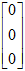，任何时候b为零向量方程组都是有解的，其实仔细想想我们知道只有当b是A中列的线性组合时这个方程才有解，也就是说当且仅当b属于A的列空间C(A)时Ax=b有解，因此矩阵的列空间非常重要，因为它能告诉我们方程什么时候有解。
矩阵A的零空间（null space）
零空间是跟列空间完全不同的子空间，A的列空间关心的是什么样的b使得Ax=b有解，而A的零空间则关心的是当b为零向量，即Ax=0时所有的解x，也就是说列空间关心的是b，而零空间关心的是b=0时的x，还是以A=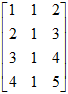为例，其零空间是什么？首先可以肯定的是它是R3的一个子空间，注意刚刚的列空间是R4的子空间，不管矩阵A是多少，其零空间N(A)一定包含零向量，在这个例子中，我们容易得到其他的解向量x为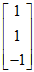，推广一下可得所有形如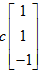的向量都在A的零空间里，这个零空间是R3中的一条两端延伸且过原点的直线。
那么零空间是向量空间吗？很显然是的，因为假设b=0时方程组有两个解x1和x2，那么它们的线性组合仍然是方程组的解，即Ax1=0，Ax2=0，那么A(c1x1+c2x2)=0，所以Ax=0的解构成一个子空间。
既然讲了矩阵的零空间是向量空间，那么我们可以看一下b不等于0时的情况，假设现有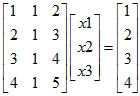，刚刚已经说过，如果随便取b，很有可能方程无解，但这里给出的b很简单，有些解一下子就能看出来，但我们不关心那些解是什么，我们关心的是这些解构成向量空间吗？假设x1和x2是两个解，很显然c1x1+c2x2不再是方程的解，因此当b不等于0时，这些解就不构成向量空间了，或者我们通过零向量也可看出这些解不构成向量空间，前面我们说所有的向量空间都必须包含零向量，不包含零向量的肯定不是向量空间，这里当x=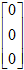时显然不能满足方程，因此这些解不构成向量空间。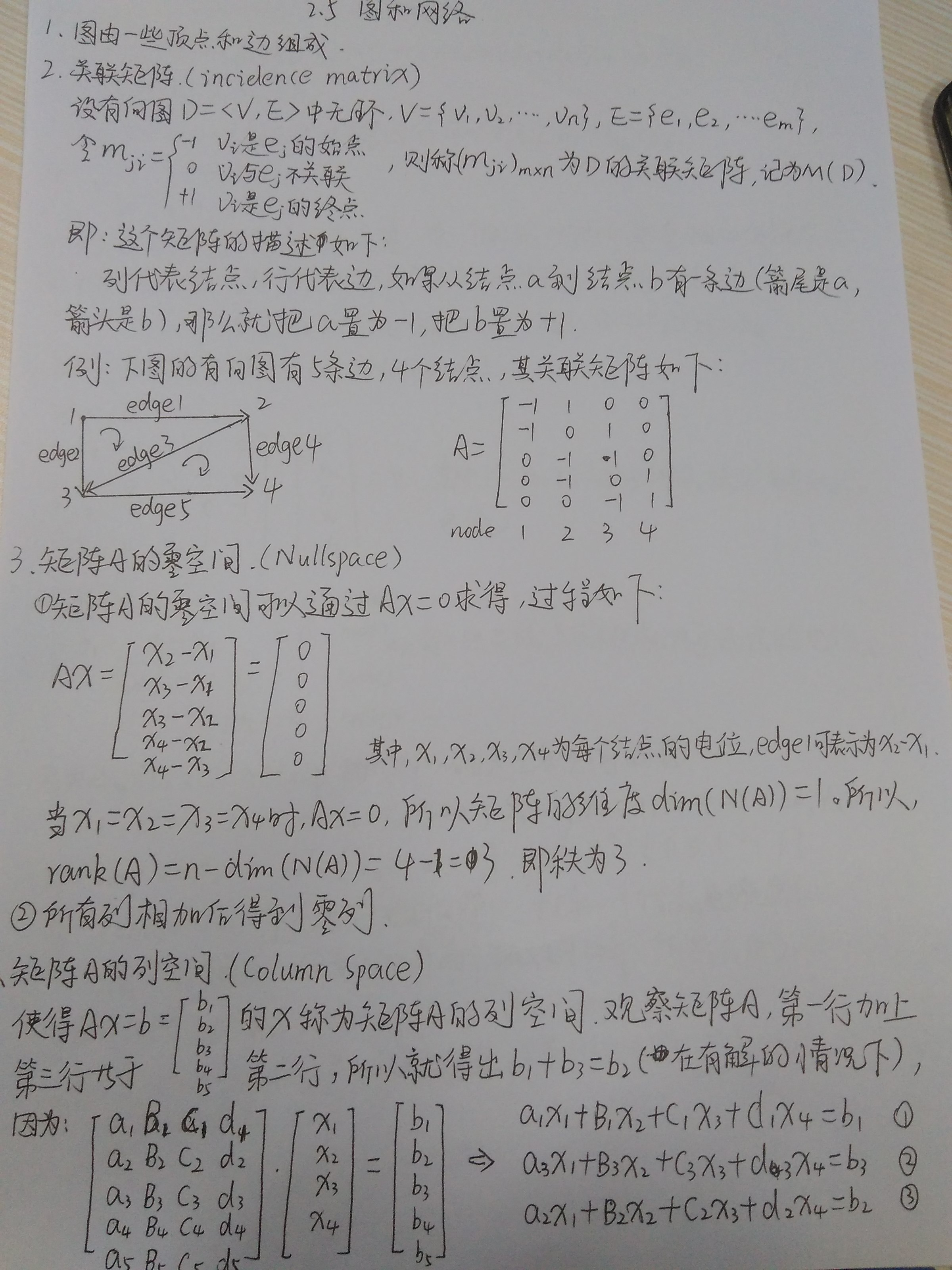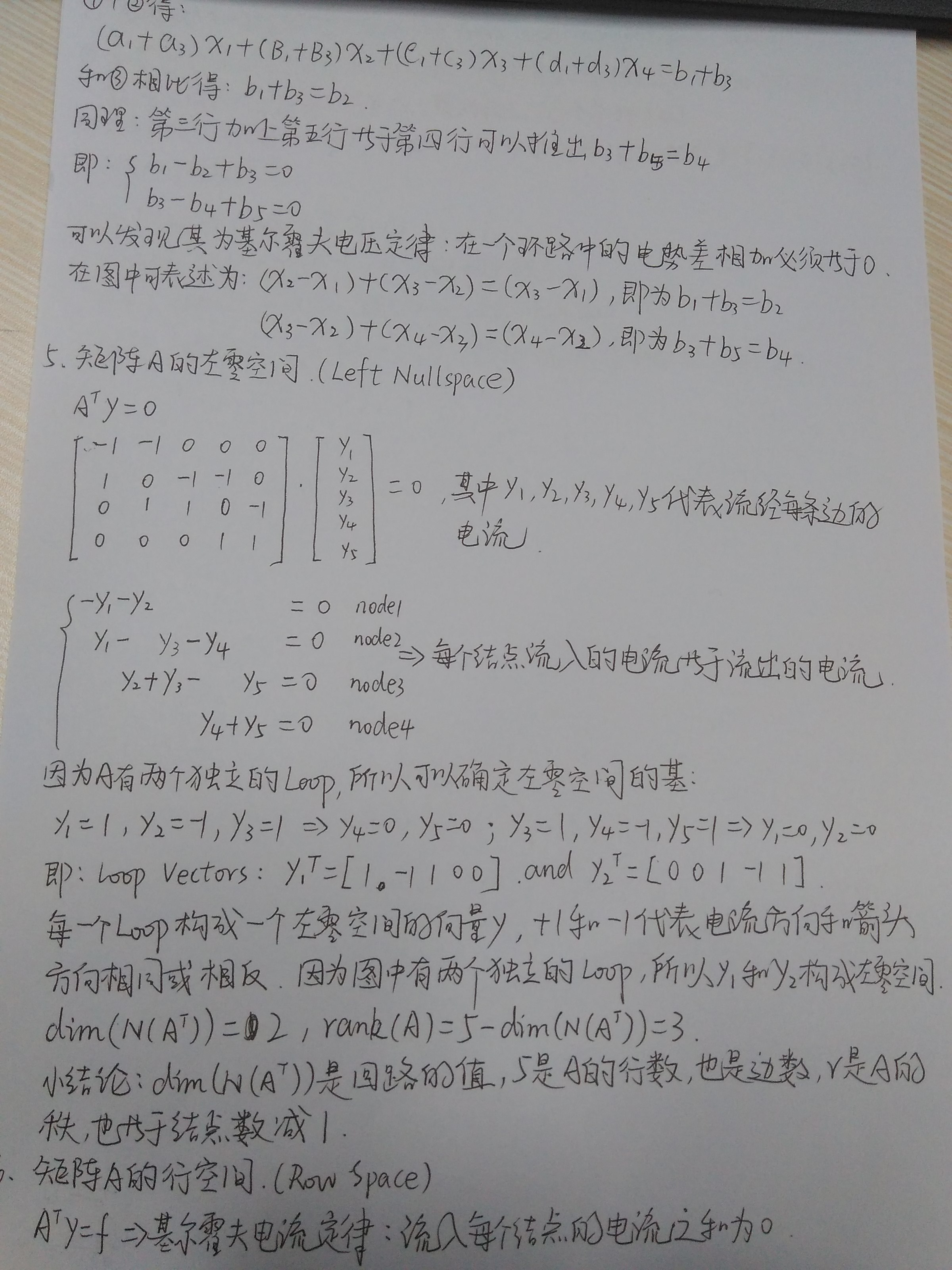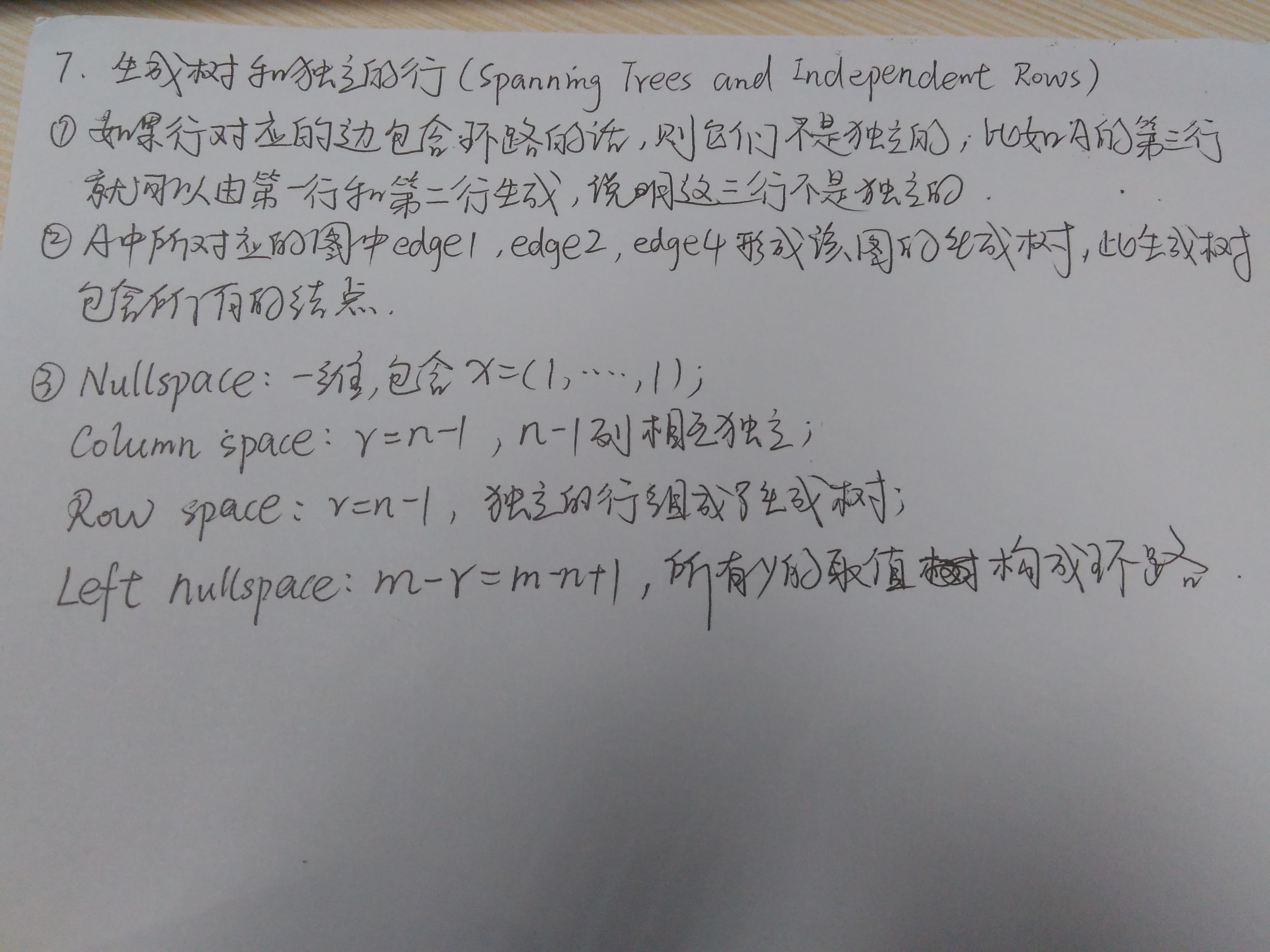参考书籍中的2.5 Graphs and Networks：Linear Algebra and its Application(4ed) Gilbert_Strang

展开全文网络
• 根据关联矩阵“mInc”返回稀疏邻接矩阵“mAdj”。 关联矩阵行必须代表边，而列必须代表顶点。 函数可以处理关联矩阵包含 -1s 的有向图，表示“进入”边，1s 表示“外出”边。matlab
• 这题首先要明白关联矩阵是怎么一回事。关联矩阵是用结点与支路的关系...于是，该有向图的关联矩阵为一个（n*b）阶的矩阵，用Aij 表示。它的每一行对应一个结点，每一列对应一条支路，它的任一元素 aij定义如下： a...
• 一、题目：算法训练 关联矩阵 问题描述 　有一个n个结点m条边的有向图...输出该图的关联矩阵，注意请勿改变边和结点的顺序。 样例输入 5 9 1 2 3 1 1 5 2 5 2 3 2 3 3 2 4 3 5 4 样例输出 1 -1 1 0 0 0 0 0 0 -1 0 0 1
• 邻接矩阵与关联矩阵均是图的不同形式的矩阵表示，他们均可代表图的拓扑结构，无论是对于无向图还是有向图它们之间均能相互转换，下列代码实现了无向图的关联矩阵与邻接矩阵之间的相互转化。 function res = trans(M,...算法 matlab
• 邻接矩阵代码稀疏因子关联矩阵节点支路关联矩阵回路关联矩阵割集关联矩阵权矩阵邻接表无向图的邻接表表示带权图的邻接表表示有向图的邻接表（出边表）有向图的逆邻接表（入边表）向邻接表Graph中插入边图的邻接表...
• ## 图,网络,关联矩阵

万次阅读 2012-06-06 20:49:59
最近看了一下MITStrang教授线性代数课，尤其是当看到图和网络...在实际应用中，可以给边加上箭头来表示电流流向，这就是一个有向图： 然后我们可以定义一个关联矩阵(Incidence Matrices)，这个矩阵描述如网络 matrix tree c
• 问题描述 　有一个n个结点m条边的有向图，请输出他的关联矩阵。 输入格式 　第一行两个整数n、m，...　输出该图的关联矩阵，注意请勿改变边和结点的顺序。 样例输入 5 9 1 2 3 1 1 5 2 5 2 3 2 3 3 2 4 3 5 4 样java
• 关联矩阵 资源限制 时间限制：1.0s 内存限制：512.0MB 问题描述 　有一个n个结点m条边的有向图，...　输出该图的关联矩阵，注意请勿改变边和结点的顺序。 样例输入 5 9 1 2 3 1 1 5 2 5 2 3 2 3 3 2 4 3 5 4 样例输出 1java 算法...